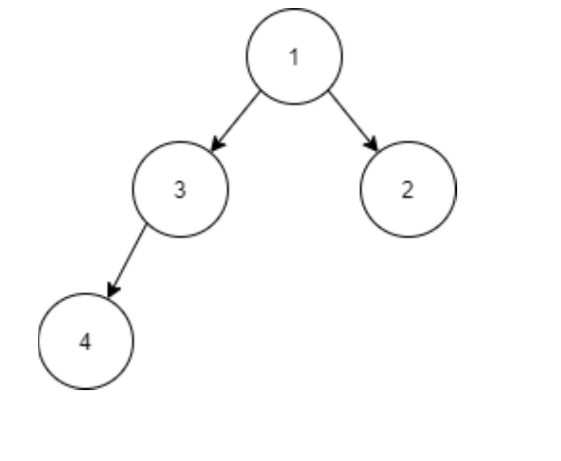New update is available. Click here to update.

# Check Whether Binary tree Is Complete

Last Updated: 25 Mar, 2021
Difficulty: Moderate

## PROBLEM STATEMENT

#### Example of a complete binary tree :##### Input Format :
``````The first line of input contains an integer 'T' representing the number of test cases. Then the test cases follow.

The only line of each test case contains elements in the level order form. The line consists of values of nodes separated by a single space. In case a node is null, we take -1 in its place.

For example, the input for the tree depicted in the below image would be :
````````````1
2 3
4 -1 5 6
-1 7 -1 -1 -1 -1
-1 -1
``````

#### Explanation :

``````Level 1 :
The root node of the tree is 1

Level 2 :
Left child of 1 = 2
Right child of 1 = 3

Level 3 :
Left child of 2 = 4
Right child of 2 = null (-1)
Left child of 3 = 5
Right child of 3 = 6

Level 4 :
Left child of 4 = null (-1)
Right child of 4 = 7
Left child of 5 = null (-1)
Right child of 5 = null (-1)
Left child of 6 = null (-1)
Right child of 6 = null (-1)

Level 5 :
Left child of 7 = null (-1)
Right child of 7 = null (-1)

The first not-null node(of the previous level) is treated as the parent of the first two nodes of the current level. The second not-null node (of the previous level) is treated as the parent node for the next two nodes of the current level and so on.

The input ends when all nodes at the last level are null(-1).
``````
##### Note :
``````The above format was just to provide clarity on how the input is formed for a given tree.

The sequence will be put together in a single line separated by a single space. Hence, for the above-depicted tree, the input will be given as:

1 2 3 4 -1 5 6 -1 7 -1 -1 -1 -1 -1 -1
``````
##### Output Format :
``````For each test case, print 1 if the given Binary Tree is a complete Binary Tree, otherwise, print 0.

Print the output of each test case in a separate line.
``````
##### Note :
``````You don’t need to print anything; It has already been taken care of. Just implement the given function.
``````
##### Constraints :
``````1 <= T <= 100
1 <= N <= 3000
1 <= data <= 10^5 and data!=-1

Where ‘N’ is the total number of nodes in the binary tree, and 'data' is the value of the binary tree node

Time Limit: 1 sec
``````## Approach 1

The idea is to observe the fact that in the Level Order Traversal of any Complete Binary Tree, all the Non-Null nodes come in succession, and all the Null nodes come after them. Using this observation, we can check whether the given binary tree is a complete binary tree. The level order traversal can be done with an approach that is similar to Breadth First Search (BFS). While traversing the Tree in level order, we will maintain a flag variable to check whether we have found a Null node till now. If, at any time, we find a Non-Null node after a Null node, then the given binary tree will not be a Complete Binary Tree, otherwise, it will always be a complete binary tree.

Steps:

1. Let q be a queue that will be used for the Level-order Traversal.
2. Insert root of the tree into the q.
3. Let isNullFound be a flag variable that checks whether we have encountered any Null node till now. Initialize it as 0.
4. While q is not empty,
• Remove a node from q, let currNode be the removed node.
• If currNode is a Null Node, then
• Set isNullFound as 1, as we have found a Null Node.
• Otherwise, if isNullFound is 1, then return 0. As we have already found a Null Node earlier, and now we found a Non-Null node. Hence, the given Binary Tree cannot be a Complete Binary Tree.
• Otherwise, add both the childs of currNode to the queue q.
5. We will return 1 if we have not returned any value till now. As we have traversed the Binary Tree and we were not able to find a Non-Null node after a Null Node. Hence, the given Tree will be a Complete Binary Tree.